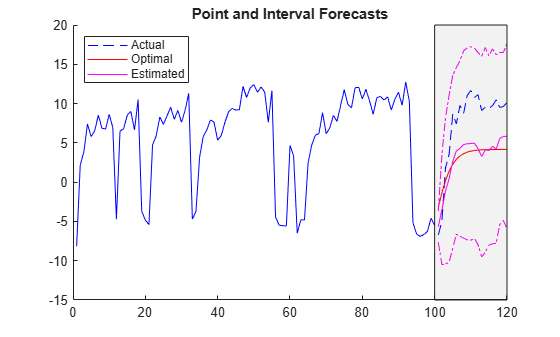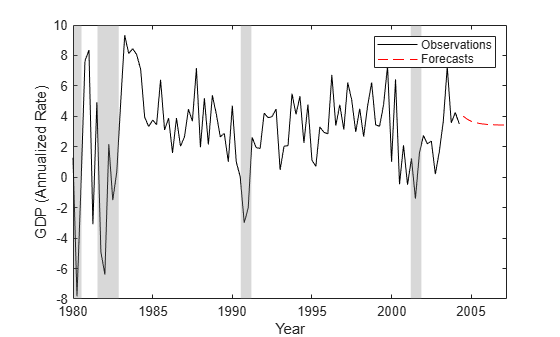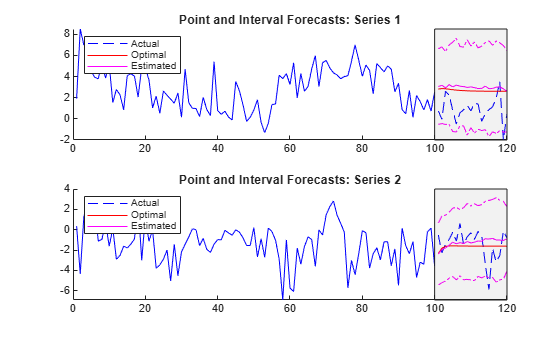# forecast

Forecast sample paths from Markov-switching dynamic regression model

## Syntax

``YF = forecast(Mdl,Y,numPeriods)``
``YF = forecast(Mdl,Y,numPeriods,Name,Value)``
``[YF,EstCov] = forecast(___)``

## Description

example

``YF = forecast(Mdl,Y,numPeriods)` returns optimal point forecasts `YF` of a fully specified Markov-switching dynamic regression model `Mdl` over a forecast horizon of length `numPeriods`. The forecasted responses represent the continuation of the response data `Y`.`

example

````YF = forecast(Mdl,Y,numPeriods,Name,Value)` uses additional options specified by one or more name-value arguments. For example, `'X',X` specifies exogenous data in the forecast horizon to evaluate regression components in the model.```

example

``[YF,EstCov] = forecast(___)` returns simulation-based forecasts `YF` and corresponding forecast error covariances `EstCov`, using any of the input argument combinations in the previous syntaxes. `

## Examples

collapse all

Forecast a response path from a two-state Markov-switching dynamic regression model for a 1-D response process. This example uses arbitrary parameter values for the data-generating process (DGP).

Create Fully Specified Model for DGP

Create a two-state discrete-time Markov chain model that describes the regime switching mechanism. Label the regimes.

```P = [0.9 0.1; 0.3 0.7]; mc = dtmc(P,'StateNames',["Expansion" "Recession"]);```

`mc` is a fully specified `dtmc` object.

For each regime, use arima to create an AR model that describes the response process within the regime. Store the submodels in a vector.

```mdl1 = arima('Constant',5,'AR',[0.3 0.2],... 'Variance',2); mdl2 = arima('Constant',-5,'AR',0.1,... 'Variance',1); mdl = [mdl1; mdl2];```

`mdl1` and `mdl2` are fully specified `arima` objects.

Create a Markov-switching dynamic regression model from the switching mechanism `mc` and the vector of submodels `mdl`.

`Mdl = msVAR(mc,mdl);`

`Mdl` is a fully specified `msVAR` object.

Simulate Response Data from DGP

forecast requires enough data before the forecast horizon to initialize the model. Simulate 120 observations from the DGP.

```rng(1); % For reproducibility y = simulate(Mdl,120);```

`y` is a 120-by-1 random path of responses.

Compute Optimal Point Forecasts

Treat the first 100 observations of the simulated response data as the presample for the forecast, and treat the last 20 observations as a holdout sample.

```idx0 = 1:100; idx1 = 101:120; y0 = y(idx0); y1 = y(idx1);```

Compute 1- through 20-step-ahead optimal point forecasts from the model.

`yf = forecast(Mdl,y0,20);`

`yf` is a 20-by-1 vector of optimal point forecasts.

Plot the simulated response data and forecasts.

```figure hold on plot(idx0,y0,'b'); h = plot(idx1,y1,'b--'); h1 = plot(idx1,yf,'r'); yfill = [ylim fliplr(ylim)]; xfill = [idx0(end) idx0(end) idx1(end) idx1(end)]; fill(xfill,yfill,'k','FaceAlpha',0.05) legend([h h1],["Actual" "Optimal"],'Location','NorthWest') title('Forecasts') hold off```Consider the model in Compute Optimal Point Forecasts.

Create Fully Specified Model for DGP

Create the fully specified Markov-switching dynamic regression model.

```P = [0.9 0.1; 0.3 0.7]; mc = dtmc(P,'StateNames',["Expansion" "Recession"]); mdl1 = arima('Constant',5,'AR',[0.3 0.2],... 'Variance',2); mdl2 = arima('Constant',-5,'AR',0.1,... 'Variance',1); mdl = [mdl1; mdl2]; Mdl = msVAR(mc,mdl);```

Simulate Response Data from DGP

`forecast` requires enough data before the forecast horizon to initialize the model. Simulate 120 observations from the DGP.

```rng(10); % For reproducibility y = simulate(Mdl,120);```

`y` is a 120-by-1 random path of responses.

Compute Monte Carlo Point Forecasts

Treat the first 100 observations of the simulated response data as the presample for the forecast, and treat the last 20 observations as a holdout sample.

```idx0 = 1:100; idx1 = 101:120; y0 = y(idx0); y1 = y(idx1);```

Compute 1- through 20-step-ahead optimal point forecasts from the model.

`yf1 = forecast(Mdl,y0,20);`

`yf2` is a 20-by-1 vector of optimal point forecasts.

Compute 1- through 20-step-ahead Monte Carlo point forecasts by returning the estimated forecast error variances.

`[yf2,estVar] = forecast(Mdl,y0,20);`

`yf2` is a 20-by-1 vector of Monte Carlo point forecasts. `estVAR` is a 20-by-1 vector of corresponding estimated forecast error variances.

Plot the simulated response data, forecasts, and 95% forecast intervals using the Monte Carlo estimates.

```figure hold on plot(idx0,y0,'b'); h = plot(idx1,y1,'b--'); h1 = plot(idx1,yf1,'r'); h2 = plot(idx1,yf2,'m'); ciu = yf2 + 1.96*sqrt(estVar); % Upper 95% confidence level cil = yf2 - 1.96*sqrt(estVar); % Lower 95% confidence level plot(idx1,ciu,'m-.'); plot(idx1,cil,'m-.'); yfill = [ylim,fliplr(ylim)]; xfill = [idx0(end) idx0(end) idx1(end) idx1(end)]; fill(xfill,yfill,'k','FaceAlpha',0.05) legend([h h1 h2],["Actual" "Optimal" "Estimated"],... 'Location',"NorthWest") title("Point and Interval Forecasts") hold off````forecast` estimates forecasts and corresponding forecast error variances by performing Monte Carlo simulation. You can adjust the number of paths to sample by specifying the `'NumPaths'` name-value pair argument. Consider the model in Compute Optimal Point Forecasts.

Create the fully specified Markov-switching dynamic regression model.

```P = [0.9 0.1; 0.3 0.7]; mc = dtmc(P,'StateNames',["Expansion" "Recession"]); mdl1 = arima('Constant',5,'AR',[0.3 0.2],... 'Variance',2); mdl2 = arima('Constant',-5,'AR',0.1,... 'Variance',1); mdl = [mdl1; mdl2]; Mdl = msVAR(mc,mdl);```

Simulate 100 observations from the DGP.

```rng(10); % For reproducibility y = simulate(Mdl,100);```

Compute 1- through 20-step-ahead Monte Carlo point forecasts by returning the estimated forecast error variances. Specify 1000 sample paths for the Monte Carlo simulation. Treat the observations `y` as the presample for the forecast.

`[yf,estVar] = forecast(Mdl,y,20,'NumPaths',1000);`

`yf` is a 20-by-1 vector of Monte Carlo point forecasts. `estVAR` is a 20-by-1 vector of corresponding estimated forecast error variances.

Consider a two-state Markov-switching dynamic regression model of the postwar US real GDP growth rate. The model has the parameter estimates presented in .

Create Markov-Switching Dynamic Regression Model

Create a fully specified discrete-time Markov chain model that describes the regime switching mechanism. Label the regimes.

```P = [0.92 0.08; 0.26 0.74]; mc = dtmc(P,'StateNames',["Expansion" "Recession"]);```

Create separate, fully specified AR(0) models for the two regimes.

```sigma = 3.34; % Homoscedastic models across states mdl1 = arima('Constant',4.62,'Variance',sigma^2); mdl2 = arima('Constant',-0.48,'Variance',sigma^2); mdl = [mdl1 mdl2];```

Create the Markov-switching dynamic regression model from the switching mechanism `mc` and the state-specific submodels `mdl`.

`Mdl = msVAR(mc,mdl);`

`Mdl` is a fully specified `msVAR` object.

`forecast` requires observations to initialize the model. Load the US GDP data set.

`load Data_GDP`

`Data` contains quarterly measurements of the US real GDP in the period 1947:Q1–2005:Q2. The period of interest in  is 1947:Q2–2004:Q2. For more details on the data set, enter `Description` at the command line.

Transform the data to an annualized rate series by:

1. Converting the data to a quarterly rate within the estimation period

2. Annualizing the quarterly rates

```qrate = diff(Data(2:230))./Data(2:229); % Quarterly rate arate = 100*((1 + qrate).^4 - 1); % Annualized rate```

The transformation drops the first observation.

Forecast US GDP Rates

Forecast the model over a 12-quarter forecast horizon. Initialize the model by supplying the annualized rate series.

```numPeriods = 12; yf = forecast(Mdl,arate,numPeriods);```

`yf` is a 12-by-1 vector of model forecasts. `yf(j)` is the `j`-step-ahead optimal point forecast.

Plot the observed annualized GDP rate from 1980 with the model forecasts.

```dates = datetime(dates(3:230),'ConvertFrom','datenum',... 'Format','yyyy:QQQ','Locale','en_US'); dt1980Q1 = datetime("1980:Q1",'InputFormat','yyyy:QQQ',... 'Locale','en_US'); % Specify US date format for 1980:Q1. idx = dates >= dt1980Q1; figure; plot(dates(idx),arate(idx),'k',... dates(end) + calquarters(1:numPeriods),yf,'r--') xlabel("Year") ylabel("GDP (Annualized Rate)") recessionplot legend("Observations","Forecasts")```Compute optimal and estimated forecasts and corresponding forecast error covariance matrices from a three-state Markov-switching dynamic regression model for a 2-D VARX response process. This example uses arbitrary parameter values for the DGP.

Create Fully Specified Model for DGP

Create a three-state discrete-time Markov chain model for the switching mechanism.

```P = [10 1 1; 1 10 1; 1 1 10]; mc = dtmc(P);```

`mc` is a fully specified `dtmc` object. `dtmc` normalizes the rows of `P` so that they sum to `1`.

For each regime, use `varm` to create a VAR model that describes the response process within the regime. Specify all parameter values.

```% Constants C1 = [1;-1]; C2 = [2;-2]; C3 = [3;-3]; % Autoregression coefficients AR1 = {}; AR2 = {[0.5 0.1; 0.5 0.5]}; AR3 = {[0.25 0; 0 0] [0 0; 0.25 0]}; % Regression coefficients Beta1 = [1;-1]; Beta2 = [2 2;-2 -2]; Beta3 = [3 3 3;-3 -3 -3]; % Innovations covariances Sigma1 = [1 -0.1; -0.1 1]; Sigma2 = [2 -0.2; -0.2 2]; Sigma3 = [3 -0.3; -0.3 3]; % VARX submodels mdl1 = varm('Constant',C1,'AR',AR1,'Beta',Beta1,'Covariance',Sigma1); mdl2 = varm('Constant',C2,'AR',AR2,'Beta',Beta2,'Covariance',Sigma2); mdl3 = varm('Constant',C3,'AR',AR3,'Beta',Beta3,'Covariance',Sigma3); mdl = [mdl1; mdl2; mdl3];```

`mdl` contains three fully specified `varm` model objects.

For the DGP, create a fully specified Markov-switching dynamic regression model from the switching mechanism `mc` and the submodels `mdl`.

`Mdl = msVAR(mc,mdl);`

`Mdl` is a fully specified `msVAR` model.

Forecast Model Ignoring Regression Component

If you do not supply exogenous data, `simulate` and `forecast` ignore the regression components in the submodels. `forecast` requires enough data before the forecast horizon to initialize the model. Simulate 120 observations from the DGP.

```rng('default'); % For reproducibility Y = simulate(Mdl,120);```

`Y` is a 120-by-2 matrix of simulated responses. Rows correspond to time points, and columns correspond to variables in the system.

Treat the first 100 observations of the simulated response data as the presample for the forecast, and treat the last 20 observations as a holdout sample.

```idx0 = 1:100; idx1 = 101:120; Y0 = Y(idx0,:); % Forecast sample Y1 = Y(idx1,:); % Holdout sample```

Compute 1- through 20-step-ahead optimal and estimated point forecasts from the model. Compute forecast error covariance matrices corresponding to the estimated forecasts.

```YF1 = forecast(Mdl,Y0,20); [YF2,EstCov] = forecast(Mdl,Y0,20);```

`YF1` and `YF2` are 20-by-2 matrices of optimal and estimated forecasts, respectively. `EstCov` is a 2-by-2-by-20 array of forecast error covariances.

Extract the forecast error variances of each response for each period in the forecast horizon.

```estVar1(:) = EstCov(1,1,:); estVar2(:) = EstCov(2,2,:);```

`estVar1` and `estVar2` are 1-by-20 vectors of forecast error variances.

Plot the data, forecasts, and 95% forecast intervals of each variable on separate subplots.

```figure subplot(2,1,1) hold on plot(idx0,Y0(:,1),'b'); h = plot(idx1,Y1(:,1),'b--'); h1 = plot(idx1,YF1(:,1),'r'); h2 = plot(idx1,YF2(:,1),'m'); ciu = YF2(:,1) + 1.96*sqrt(estVar1'); % Upper 95% confidence level cil = YF2(:,1) - 1.96*sqrt(estVar1'); % Lower 95% confidence level plot(idx1,ciu,'m-.'); plot(idx1,cil,'m-.'); yfill = [ylim,fliplr(ylim)]; xfill = [idx0(end) idx0(end) idx1(end) idx1(end)]; fill(xfill,yfill,'k','FaceAlpha',0.05) legend([h h1 h2],["Actual" "Optimal" "Estimated"],... 'Location',"NorthWest") title("Point and Interval Forecasts: Series 1") hold off subplot(2,1,2) hold on plot(idx0,Y0(:,2),'b'); h = plot(idx1,Y1(:,2),'b--'); h1 = plot(idx1,YF1(:,2),'r'); h2 = plot(idx1,YF2(:,2),'m'); ciu = YF2(:,2) + 1.96*sqrt(estVar2'); % Upper 95% confidence level cil = YF2(:,2) - 1.96*sqrt(estVar2'); % Lower 95% confidence level plot(idx1,ciu,'m-.'); plot(idx1,cil,'m-.'); yfill = [ylim,fliplr(ylim)]; xfill = [idx0(end) idx0(end) idx1(end) idx1(end)]; fill(xfill,yfill,'k','FaceAlpha',0.05) legend([h h1 h2],["Actual" "Optimal" "Estimated"],... 'Location',"NorthWest") title("Point and Interval Forecasts: Series 2") hold off```Forecast Model Including Regression Component

Simulate exogenous data for the three regressors by generating 120 random observations from the 3-D standard Gaussian distribution.

`X = randn(120,3);`

Simulate 120 observations from the DGP. Specify the exogenous data for the regression component.

```rng('default') Y = simulate(Mdl,120,'X',X);```

Treat the first 100 observations of the simulated response and exogenous data as the presample for the forecast, and treat the last 20 observations as a holdout sample.

```idx0 = 1:100; idx1 = 101:120; Y0 = Y(idx0,:); Y1 = Y(idx1,:); X1 = X(idx1,:);```

Compute 1- through 20-step-ahead optimal and estimated point forecasts from the model. Compute forecast error covariance matrices corresponding to the estimated forecasts. Specify the forecast-period exogenous data for the regression component.

```YF1 = forecast(Mdl,Y0,20,'X',X1); [YF2,EstCov] = forecast(Mdl,Y0,20,'X',X1); estVar1(:) = EstCov(1,1,:); estVar2(:) = EstCov(2,2,:);```

Plot the data, forecasts, and 95% forecast intervals of each variable on separate subplots.

```figure subplot(2,1,1) hold on plot(idx0,Y0(:,1),'b'); h = plot(idx1,Y1(:,1),'b--'); h1 = plot(idx1,YF1(:,1),'r'); h2 = plot(idx1,YF2(:,1),'m'); ciu = YF2(:,1) + 1.96*sqrt(estVar1'); % Upper 95% confidence level cil = YF2(:,1) - 1.96*sqrt(estVar1'); % Lower 95% confidence level plot(idx1,ciu,'m-.'); plot(idx1,cil,'m-.'); yfill = [ylim,fliplr(ylim)]; xfill = [idx0(end) idx0(end) idx1(end) idx1(end)]; fill(xfill,yfill,'k','FaceAlpha',0.05) legend([h h1 h2],["Actual" "Optimal" "Estimated"],... 'Location',"NorthWest") title("Point and Interval Forecasts: Series 1") hold off subplot(2,1,2) hold on plot(idx0,Y0(:,2),'b'); h = plot(idx1,Y1(:,2),'b--'); h1 = plot(idx1,YF1(:,2),'r'); h2 = plot(idx1,YF2(:,2),'m'); ciu = YF2(:,2) + 1.96*sqrt(estVar2'); % Upper 95% confidence level cil = YF2(:,2) - 1.96*sqrt(estVar2'); % Lower 95% confidence level plot(idx1,ciu,'m-.'); plot(idx1,cil,'m-.'); yfill = [ylim,fliplr(ylim)]; xfill = [idx0(end) idx0(end) idx1(end) idx1(end)]; fill(xfill,yfill,'k','FaceAlpha',0.05) legend([h h1 h2],["Actual" "Optimal" "Estimated"],... 'Location',"NorthWest") title("Point and Interval Forecasts: Series 2") hold off```## Input Arguments

collapse all

Fully specified Markov-switching dynamic regression model, specified as an `msVAR` model object returned by `msVAR` or `estimate`. Properties of a fully specified model object do not contain `NaN` values.

Response data that provides initial values for the forecasts, specified as a `numObs`-by-`numSeries` numeric matrix.

`numObs` is the sample size. `numSeries` is the number of response variables (`Mdl.NumSeries`).

Rows correspond to observations, and the last row contains the latest observation. Columns correspond to individual response variables.

The forecasts `YF` represent the continuation of `Y`.

Data Types: `double`

Forecast horizon, or the number of time points in the forecast period, specified as a positive integer.

Data Types: `double`

### Name-Value Arguments

Specify optional pairs of arguments as `Name1=Value1,...,NameN=ValueN`, where `Name` is the argument name and `Value` is the corresponding value. Name-value arguments must appear after other arguments, but the order of the pairs does not matter.

Before R2021a, use commas to separate each name and value, and enclose `Name` in quotes.

Example: `'X',X` uses the matrix `X` as exogenous data in the forecast horizon to evaluate regression components in the model.

Initial state probabilities from which to forecast, specified as the comma-separated pair consisting of `'S0'` and a nonnegative numeric vector of length `numStates`. `S0` corresponds to the end of the response data sample `Y`.

`forecast` normalizes `S0` to produce a distribution.

By default, `forecast` applies `smooth` to `Y` using default settings, then sets `S0` to the terminal distribution of states in the data.

Example: `'S0',[0.2 0.2 0.6]`

Example: `'S0',[0 1]` specifies state 2 as the initial state.

Data Types: `double`

Predictor data in the forecast horizon used to evaluate regression components in all submodels of `Mdl`, specified as the comma-separated pair consisting of `'X'` and a numeric matrix or a cell vector of numeric matrices. The first row of `X` contains observations in the period after the period represented by the last observation in `Y`.

To use a subset of the same predictors in each state, specify `X` as a matrix with `numPreds` columns and at least `numPeriods` rows. Columns correspond to distinct predictor variables. Submodels use initial columns of the associated matrix, in order, up to the number of submodel predictors. The number of columns in the `Beta` property of `Mdl.SubModels(j)` determines the number of exogenous variables in the regression component of submodel `j`. If the number of rows exceeds `numPeriods`, then `forecast` uses the earliest observations.

To use different predictors in each state, specify a cell vector of such matrices with length `numStates`.

By default, `forecast` ignores the regression components in `Mdl`.

Data Types: `double`

Number of sample paths to generate for the simulation, specified as the comma-separated pair consisting of `'NumPaths'` and a positive integer. If `forecast` returns only `YF`, it ignores `NumPaths`.

Example: `'NumPaths',1000`

Data Types: `double`

## Output Arguments

collapse all

Point forecasts, returned as a `numPeriods`-by-`numSeries` numeric matrix.

If `forecast` returns only `YF`, then point forecasts are optimal. Otherwise, `forecast` uses Monte Carlo simulation to estimate the point forecasts.

Forecast error covariances, returned as a numeric column vector or numeric array.

If the submodels `Mdl.SubModels` represent univariate ARX models, `EstCov` is a `numPeriods`-by-1 vector. If `Mdl.SubModels` represent multivariate VARX models, `EstCov` is a `numSeries`-by-`numSeries`-by-`numPeriods` array.

`forecast` performs Monte Carlo simulation to compute `EstCov`.

## Algorithms

Hamilton  provides a statistically optimal, one-step-ahead point forecast `YF` for a Markov-switching dynamic regression model. `forecast` computes `YF` iteratively to the forecast horizon when called with a single output. Nonlinearity of the Markov-switching dynamic regression model leads to nonnormal forecast errors, which complicate interval and density forecasts . As a result, `forecast` switches to Monte Carlo methods when it returns `EstCov`.

 Chauvet, M., and J. D. Hamilton. "Dating Business Cycle Turning Points." In Nonlinear Analysis of Business Cycles (Contributions to Economic Analysis, Volume 276). (C. Milas, P. Rothman, and D. van Dijk, eds.). Amsterdam: Emerald Group Publishing Limited, 2006.

 Hamilton, J. D. "Analysis of Time Series Subject to Changes in Regime." Journal of Econometrics. Vol. 45, 1990, pp. 39–70.

 Krolzig, H.-M. Markov-Switching Vector Autoregressions. Berlin: Springer, 1997.